# PSEB 10th Class Maths Solutions Chapter 3 Pair of Linear Equations in Two Variables Ex 3.1

Punjab State Board PSEB 10th Class Maths Book Solutions Chapter 3 Pair of Linear Equations in Two Variables Ex 3.1 Textbook Exercise Questions and Answers.

## PSEB Solutions for Class 10 Maths Chapter 3 Pair of Linear Equations in Two Variables Ex 3.1

Question 1.
Aftab tells his daughter, “Seven years ago I was seven times as old as you were then. Also, three years from now, I shall
be three times as old as you will be” (Isn’t this interesting ?). Represent this situation algebraically and graphically.
Solution:
Let Aftabs present age = x years
and Aftab’s daughter’s present age = y years
Algebraical-Situation
According to 1st condition,
x – 7 = 7(y – 7)
or x – 7 = 7y – 49
or x – 7y + 42 = 0
According to 2nd condition,
x + 3 = 3(y + 3)
or x + 3 = 3y + 9
or x – 3y – 6 = 0
∴ Pair of Line’ar Equation in two variables are
x – 7y + 42 = 0
and x – 3y – 6 = 0

Graphical – Situation:
x – 7y + 42 = 0
x – 3y – 6 = 0

x = 7y – 42 ………….(1)
x = 3y + 6 …………..(2)
Putting y = 5 in (1), we get
Putting y = 0 in (2), we get

x = 7 × 5 – 42 = 35 – 42
x = -7
Putting y = 6 in (1), we get
x = 7 × 6 – 42 = 42 – 42 = 0
Putting y = 7 in (1), we get:
x = 7 × 7 – 42 = 49 – 42 = 7

Table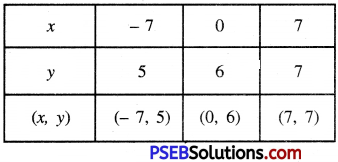Plotting the points A (-7, 5), B (0, 6), C (7, 7) and drawing a line joining them, we get the graph of the equation x – 7y + 42 = 0
x = 3 × 0 + 6
x = 0 + 6 = 6
Putting y = 3 in (2), we get:
x = 3 × 3 + 6
= 9 + 6 = 15
Putting y = -2 in (2), we get:
x = 3 × -2 + 6
= -6 + 6 = 0

Table: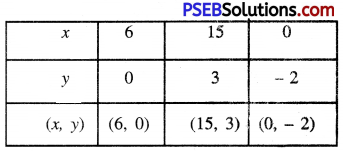Plotting the points D (6, 0), E (15, 3), F (0, -2) and drawing a line joining them, we get the graph of the equation x – 3y – 6 = 0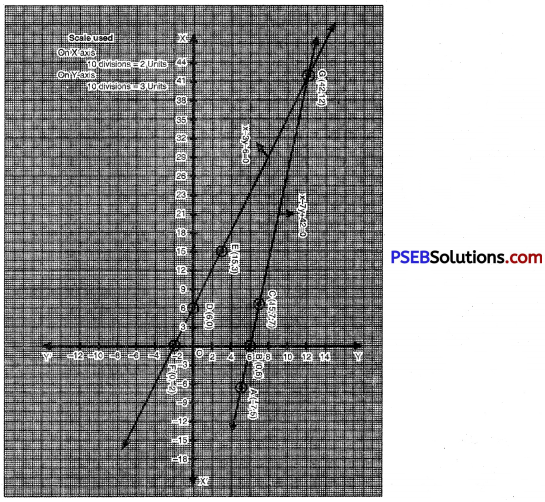From the graph it is clear that the two lines intersect at G (42, 12).
Hence, x = 42 and y = 12 is the solution of given pair of linear equations.Question 2.
The coach of a cricket team buys 3 bats and 6 balls for 3900. Later, she buys another bat and 3 more balls of the same kind for 1300. Represent this situation algebraically and geometrically. [Pb. 2019, Set-A, B, C]
Solution:
Let cost of one bat = x
Cost of one hail = y
Algehraical – Situation
According to 1st condition,
3x + 6y = 3900
or x + 2y = 1300
According to 2nd condition,
1x + 3y = 1300
∴ Pair of linear equations in two variables are:
x + 2y = 13001
and x + 3y = 1300

Graphical – Situation:
x + 2y = 1300
x = 1300 – 2y …………..(1)
Putting y = 0 in (1), we get
x = 1300 – 2 × 0
x= 1300
Putting y = 500 in (1), we get :
= 1300 – 2 × 500
= 1300 – 1000 = 300
Putting y = 650 in (1), we get :
x = 1300 – 2 × 650
1300 – 1300 = 0

Table: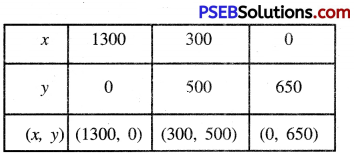Plotting the points A (1300, 0), B (300, 500) and C (0, 650) drawing a line joining them we get the graph of the equation x + 2y = 1300.
x + 3y = 1300
x = 1300 – 3y …………….(2)
Putting y = 0 in (2), we get :
x = 1300 – 3 × 0 = 1300
Putting y = 500 in (2), we get :
x = 1300 – 3 × 500
= 1300 – 1500 = – 200
Putting y = 300 in (2), we get :
x = 1300 – 3 × 300
= 1300 – 900 = 400

Table: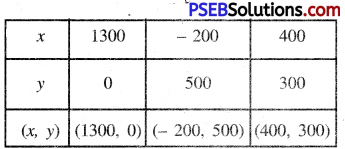Plotting the points A (1300, 0), E (-200, 500), F (400, 300) and drawing a line joining them we get the graph of the
equation.
x + 3y = 1300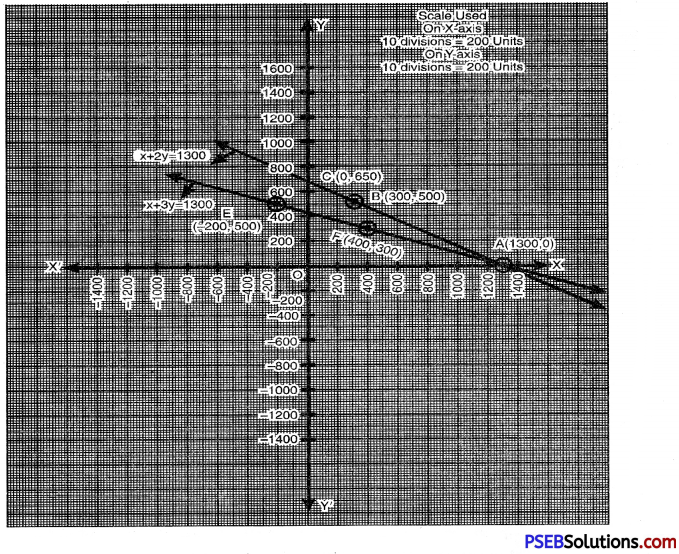From the graph it is clear that the two lines intersect at A (1300, 0).
Hence x = 1300 and y = 0 is the solution of given pair of linear equations.Question 3.
The cost of 2 kg of apples and 1 kg of grapes on a day was found to be 160. After a month, the cost of 4 kg of apples and 2 kg of grapes is ₹ 300. Represent the situation algebraically and geometrically.
Solution:
Let cost of 1 kg apples = ₹ x
Cost of 1 kg grapes = ₹ y
Algebraical – Situation
According to 1st condition,
2x + 1y = 160
According to 2nd condition,
4x + 2y = 300
∴ Pair of linear equations in two variables
2x + y = 160
and 4x + 2y = 300
Graphical – Situation
2x + y = 160
2x = 160 – y
x = $$\frac{160-y}{2}$$ ……………(1)
Putting y = 0 in (1), we get :
x = $$\frac{160-0}{2}=\frac{160}{2}$$ = 80
Putting y = 60 in (1), we get :
x = $$\frac{160-60}{2}=\frac{100}{2}$$ = 50
Putting y = 160 in (1), we get :
x = $$\frac{160-160}{2}=\frac{0}{2}$$ = 0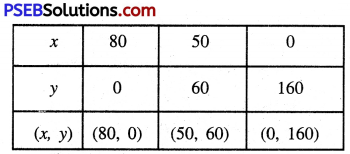Plotting the points A (80, 0), B (50, 60), C (0, 160) and drawing a line joining them, we get the graph of the equation 2x + y = 160
Now 4x + 2y = 300
or 2x + y = 150
2x = 150 – y
x = $$\frac{160-y}{2}$$ …………(2)
Putting y = 0 in (2), we get:
x = $$\frac{160-0}{2}=\frac{150}{2}$$ = 75
Putting y = 50 in (2), we get:
x = $$\frac{150-50}{2}=\frac{100}{2}$$ = 50
Putting y = 150 in (2), we get:
x = $$\frac{150-150}{2}=\frac{0}{2}$$ = 0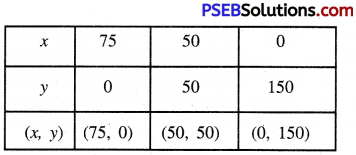Plotting the points D (75, 0), E (50, 50), F (0, 150) and drawing a line joining them, we get the graph of equation
4x + 2y = 300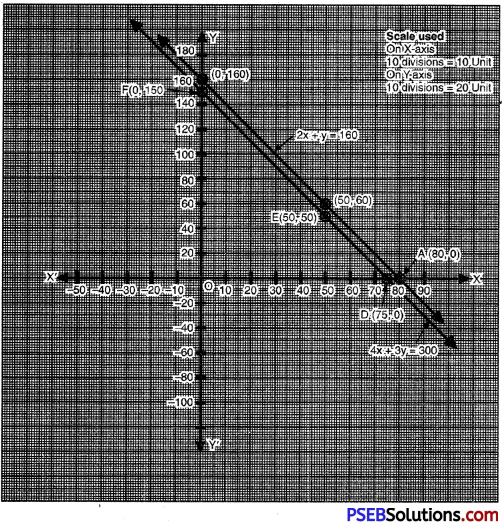From the graph, it is clear that the two lines do not intersect anywhere i.e. they are parallel.